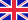•# Mathematical Methods of Statistics (PMS-9), Volume 9

Autor: Harald Cramér

In this classic of statistical mathematical theory, Harald Cramér joins the two major lines of development in the field: while British and American statisticians were developing the science of statistical inference, French and Russian probabilitists transformed... Viac o knihe

Na objednávku, dodanie 2-4 týždne

107.89 €

bežná cena: 122.60 €

## O knihe

In this classic of statistical mathematical theory, Harald Cramér joins the two major lines of development in the field: while British and American statisticians were developing the science of statistical inference, French and Russian probabilitists transformed the classical calculus of probability into a rigorous and pure mathematical theory. The result of Cramér's work is a masterly exposition of the mathematical methods of modern statistics that set the standard that others have since sought to follow.

For anyone with a working knowledge of undergraduate mathematics the book is self contained. The first part is an introduction to the fundamental concept of a distribution and of integration with respect to a distribution. The second part contains the general theory of random variables and probability distributions while the third is devoted to the theory of sampling, statistical estimation, and tests of significance.

• Vydavateľstvo: Princeton University Press
• Rok vydania: 1999
• Formát: Paperback
• Rozmer: 234 x 156 mm
• Jazyk: Anglický jazyk
• ISBN: 9780691005478

Generuje redakčný systém BUXUS CMS spoločnosti ui42.